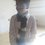# An amazing identity!

Hello! I'd like to share with you an identity I found in one of my old question papers. It says that $\text{If}~x+\frac{1}{x}=2\cos\theta,~\text{then}~x^n+\frac{1}{x^n}=2\cos n\theta$ $$\forall n\in\mathbb{Z}$$. It can be proved very easily. The proof goes like this.

Let $x=\cos\theta+i\sin\theta$. Then $\frac{1}{x}=\frac{1}{cos\theta+i\sin\theta}=\cos\theta-i\sin\theta$

Now, $(\cos\theta\pm i\sin\theta)^n$

$= (1,\pm\theta)^n~~~~~~(\text{polar}~\text{coordinates})$

$= (1^n,\pm n\theta)$

$= (1,\pm n\theta)$

$=\cos n\theta\pm i\sin n\theta$

$\therefore x^{n}+\frac{1}{x^{n}}$

$=\cos n\theta+i\sin n\theta + \cos n\theta-i\sin n\theta$

$= 2\cos n\theta$

This identity comes very handy in solving sums like this, this and other sums which I've collected in this set. If you have any other problems which can be solved by this identity, comment a link to it, and I'll add it to the set.

The only problem is that its use is very limited. It requires $\frac{\left\lvert x+\frac{1}{x} \right\rvert}{2} <1$, as that is the condition for $2\cos\theta$. If you have any other additions to this identity, or methods to cross these limitations, comment! This wiki may come handy. Maybe it could help cross these limitations.Note by Omkar Kulkarni
6 years, 4 months ago

This discussion board is a place to discuss our Daily Challenges and the math and science related to those challenges. Explanations are more than just a solution — they should explain the steps and thinking strategies that you used to obtain the solution. Comments should further the discussion of math and science.

When posting on Brilliant:

• Use the emojis to react to an explanation, whether you're congratulating a job well done , or just really confused .
• Ask specific questions about the challenge or the steps in somebody's explanation. Well-posed questions can add a lot to the discussion, but posting "I don't understand!" doesn't help anyone.
• Try to contribute something new to the discussion, whether it is an extension, generalization or other idea related to the challenge.
• Stay on topic — we're all here to learn more about math and science, not to hear about your favorite get-rich-quick scheme or current world events.

MarkdownAppears as
*italics* or _italics_ italics
**bold** or __bold__ bold
- bulleted- list
• bulleted
• list
1. numbered2. list
1. numbered
2. list
Note: you must add a full line of space before and after lists for them to show up correctly
paragraph 1paragraph 2

paragraph 1

paragraph 2

[example link](https://brilliant.org)example link
> This is a quote
This is a quote
    # I indented these lines
# 4 spaces, and now they show
# up as a code block.

print "hello world"
# I indented these lines
# 4 spaces, and now they show
# up as a code block.

print "hello world"
MathAppears as
Remember to wrap math in $$ ... $$ or $ ... $ to ensure proper formatting.
2 \times 3 $2 \times 3$
2^{34} $2^{34}$
a_{i-1} $a_{i-1}$
\frac{2}{3} $\frac{2}{3}$
\sqrt{2} $\sqrt{2}$
\sum_{i=1}^3 $\sum_{i=1}^3$
\sin \theta $\sin \theta$
\boxed{123} $\boxed{123}$

## Comments

Sort by:

Top Newest

And yes, there should have been an extra condition specified that $n \in \mathbb{Z}$.

By the way, nice note.

- 6 years, 3 months ago

Log in to reply

Yeah, you're right. I added that. Thanks!

- 6 years, 3 months ago

Log in to reply

Thanks @Omkar Kulkarni for sharing this note :)

- 6 years, 4 months ago

Log in to reply

Welcome :D

- 6 years, 4 months ago

Log in to reply

×

Problem Loading...

Note Loading...

Set Loading...